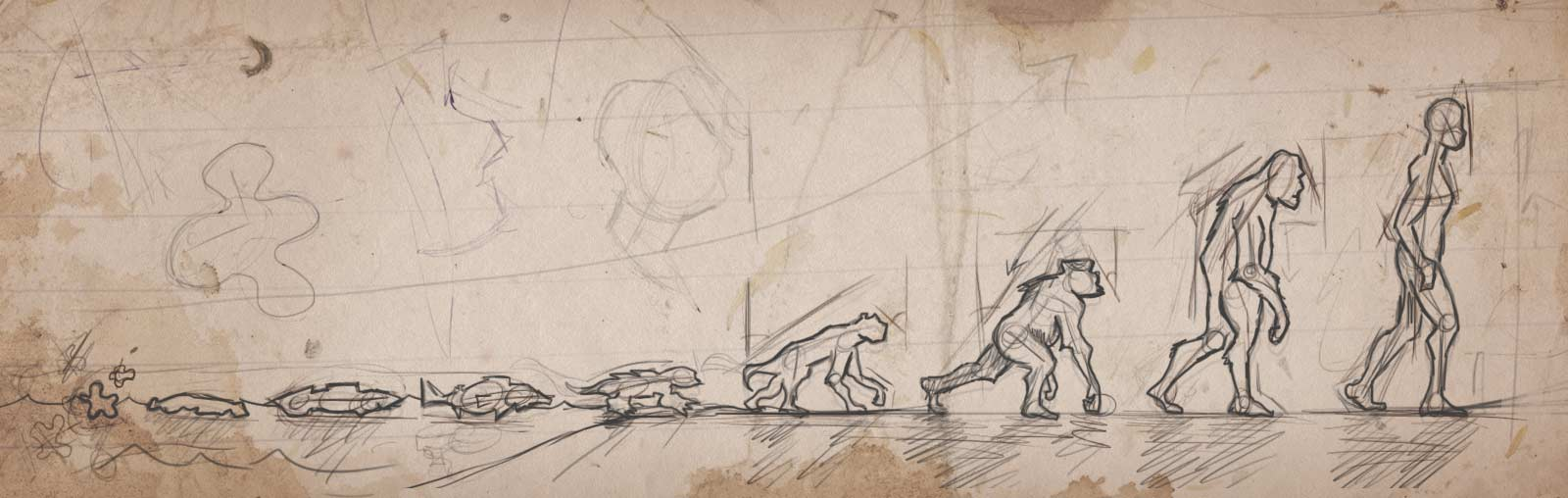Evolution, Chance, and Creation

on June 1, 1981
Share:

Originally published in Creation 4, no 2 (June 1981): 22-23.

When we toss a coin, we expect it to land showing either a head or tail.

50:50

We say from experience that the probability of the coin landing “heads” is one half, or we can say “tails” has a 50% chance of showing up. We also know from experience that this does not mean when we throw a head first, the next throw will be a tail. It simply means that if we keep tossing coins long enough, then half the time the coin lands, it will show heads, and half the time tails.

However, even in the idea of “1/2 heads” are some assumptions or beliefs which few of us bother to check when throwing coins. We take it for granted that the coin weighs the same on both head and tail sides, so it gives an unbiased result. We rarely check to see if the coin has both a head and a tail, even though rare double headed coins do exist.

An interesting situation arises when two separate events occur at the same time, e.g. tossing a coin and throwing a die. (Most of you will call it a dice, but that is plural for more than one die.) If we ask what is the “chance” of throwing a head and a 6 at the same time, a simple look at all the possible results of throwing coins and dice will show the answer.

Since the coin has two sides and only one head, the possibility of a head is 1/2.

Since the die has six sides and only one face with 6 on it, the possibility of six is 1/6.

The only trouble is that half of the times the die lands showing a 6, the coin will show a tail, the other half of the times we throw a 6, the coin would show a head. So the probability of throwing the head and the 6 together, must be one half of the sixes, or put mathematically, 1/2 x 1/6. This, of course, is 1/12. Again we must remember that this does not mean one in every 12 throws, but if you throw for long enough, 1/12 of all throws would have both a 6 and a head.1 Puts one off gambling somewhat!

Can “chance” count to 10?

Let us extend this idea further. (A problem for any Grade 10 math’s class.) Suppose we have a bucket in which are placed ten (10) identical discs, each numbered from 1–10. The question is: Can chance methods enable us to count from 1 to 10? If only one disc is to be selected from the bucket, noted and replaced, and we require disc 1 first, disc 2 second, etc. in the correct sequence from 1–10, what is the probability of selecting all ten discs in order?

The maths are relatively easy. Since there is only one disc numbered 1, there can be only one chance in ten (1/10) of selecting it. After we replace the first disc, the chance of selecting the disc number 2 is the same—1/10. In fact, every separately numbered disc has one chance in ten of being selected. The probability of selecting the first one followed by the second one in correct order must be 1/10 x 1/10 or 1/100. To select all 10 in the right order the probability is 1/10 x 1/10 x 1/10 x 1/10 x 1/10 x 1/10 x 1/10 x 1/10 x 1/10 x 1/10 or (1/10)10. This means that you would select the right order only once in 10 billion attempts. Put another way “chance” requires 10 billion attempts, on the average, to count from 1 to 10.

The theory of evolution

Further tests exist to measure how efficient chance is at producing design. The following is fascinating. The question is: What is the expected probability for chance to spell the phrase—“the theory of evolution”? This phrase by chance would involve the random selection and sequencing of letters and spaces in the correct order. Each letter from the alphabet plus one space (totaling 27 possible selections) has one chance in 27 of being selected. There are 20 letters plus 3 spaces in the phrase—“the theory of evolution.” Therefore “chance” will, on the average, spell the given phrase correctly only once in (27)23 outcomes!

This computes to only one success in a mind boggling 8.3 hundred quadrillion, quadrillion attempts (8.3x1032) (gasp!). Suppose “chance” uses a machine which removes, records and replaces all the letters randomly at the fantastic speed of one billion per microsecond (one quadrillion per second)! On average the phrase would happen once in 25 billion years by this random method. If, as evolutionists would have us believe, the earth has been in existence for approximately 5 billion years, then “chance” could take five times this time to spell out its own success, even at this phenomenal rate of experimentation. And this phrase is infinitely simpler than the smallest life form, and children of average intelligence could perform this same spelling task within a minute or so.

Amended 7 October 2004.

Footnotes

1. Statisticians have made this into a rule called the Multiplication Rule of Probability. This states that the chance of several independent results occurring at once is found by multiplying the mathematical probabilities of obtaining the individual results.